StatLect

# Standard deviation

Standard deviation is a measure of how much the realizations of a random variable are dispersed around its mean. It is equal to the square root of variance.## Definition

A precise definition follows.

Definition Letbe a random variable and let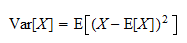be its variance. The standard deviation ofis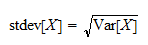The standard deviation is usually denoted byor by.

## Interpretation

Standard deviation is often deemed easier to interpret than variance because it is expressed in the same units as the random variable.

For example, ifis the height of an individual extracted at random from a population, measured in inches, then the deviation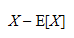is also expressed in inches.

However, the squared deviation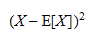and the varianceare expressed in squared inches, which makes variance hard to interpret.

Instead, the standard deviation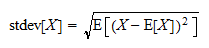is again expressed in inches. Therefore, it is easier to interpret.

## Sample standard deviation

Letbe a sample of observations having sample mean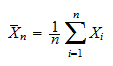and sample variance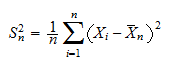The square root of the sample varianceis usually called sample standard deviation.

However, it is sometimes also simply called standard deviation, which might create confusion (sample or population?).

## Corrected standard deviation

Note thatis the biased sample variance.

An alternative is to use the unbiased sample variance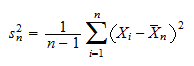The square rootis often called corrected sample standard deviation.

When the observationsare independent and have the same mean and variance,is an unbiased estimator of.

However, by Jensen's inequality,is not an unbiased estimator of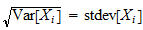.

This is the reason whycannot be called unbiased sample standard deviation and it is instead called corrected sample standard deviation.

## Standard error

The standard deviation of an estimator (e.g., the OLS estimator) is often called standard error.

## More details

More details about the standard deviation can be found in the lecture entitled Variance.

## Keep reading the glossary

Previous entry: Size of a test

Next entry: Stationary sequence